# Math in Focus Grade 4 Chapter 4 Practice 3 Answer Key Line Graphs

Go through the Math in Focus Grade 4 Workbook Answer Key Chapter 4 Practice 3 Line Graphs to finish your assignments.

## Math in Focus Grade 4 Chapter 4 Practice 3 Answer Key Line Graphs

Use the data in the line graphs to answer each question.
The line graph shows the change in Rodney’s weight over a few years.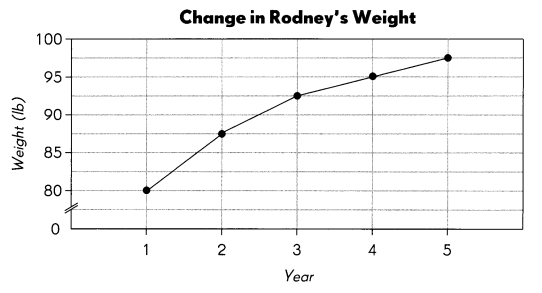Example
What was Rodney’s weight in the second year?
87.5 lb
In which year was the increase in Rodney’s weight the greatest? Between the first anti second yearQuestion 1.
What was Rodney’s weight in the
a. fourth year? ___
95 lb.

Explanation:
Rodney’s weight in the fourth year is 95 lb.

b. fifth year? ___
97.5 lb.

Explanation:
Rodney’s weight in the fifth year is 97.5 lb.

c. What was the increase in Rodney’s weight between these two years? ____

Question 2.
In which year was the increase in Rodney’s weight 5 lb?
3rd year.

Explanation:
In the 3rd year, the increase in Rodney’s weight was 5 lb which is from 87.5 lb to 92.5 lb.

The line graph shows the temperature of an object being heated over five hours.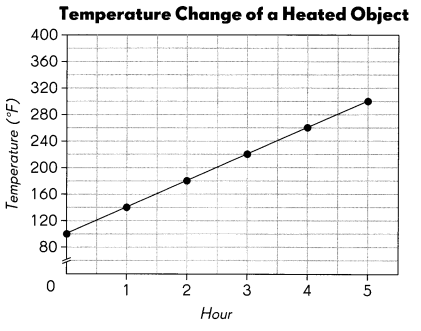Question 3.
What was the temperature of the object in the second hour? ___
180°F.

Explanation:
The temperature of the object in the second hour is 180°F.

Question 4.
What was the temperature of the object in the fourth hour? ____
260°F.

Explanation:
The temperature of the object in the fourth hour is 260°F.

Question 5.
What was the increase in temperature between the second hour and the fourth hour? ____
80°F.

Explanation:
The increase in temperature between the second hour and the fourth hour will be 260°F.-180°F. which is 80°F.

Use the data from the graph to complete the table.

Question 6.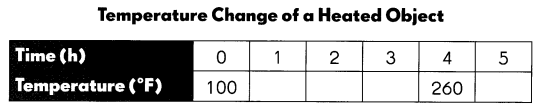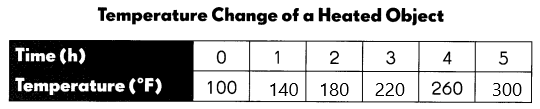Question 7.
Did the temperature increase by the same amount every hour?
Yes.

Explanation:
Yes, the temperature increase by the same amount every hour.

The line graph shows the change in height while Ali was climbing a mountain.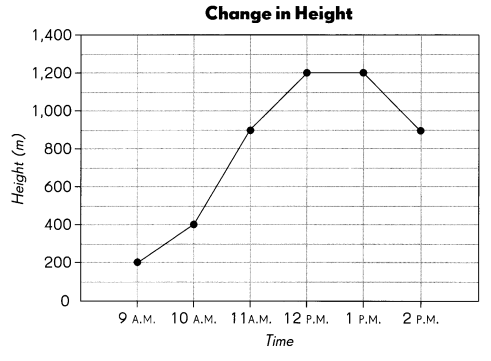Question 8.
At what time was Ali at a height of 200 meters? ____
9 A.M.

Explanation:
At 9 A.M. was Ali at a height of 200 meters.

Question 9.
At what height was Ali at 2 P.M.? ____
900 meters.

Explanation:
At 2 P.M. Ali’s height is 900 meters.

Question 10.
During which hour was the increase in height the greatest? ____
12 P.M. and 1 P.M.

Explanation:
At 12 P.M. and 1 P.M. hours the increase in height the greatest.

Question 11.
When did Ali take a break from climbing? ____
12 P.M.

Explanation:
At 12 P.M. Ali took a break from climbing.

Question 12.
Explain what happened from 1 P.M. to 2 P.M.
Height was decreasing.

Explanation:
From 1 P.M. to 2 P.M. the height was decreasing from 1,200 meters to 900 meters.

Choose an appropriate graph to display the data. Write bar graph, line graph, or picture graph. Explain your choice.

Question 13.
Anna recorded the rainfall amounts (in centimeters) in each month from January to June.